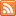# Activity 3: Simulations and Tools CategoryJava applets, interactive simulations, and other statistical tools.

## Interactive Applets – Linear Regression

Published: Dec 25th, 2012

Try these interactive demonstrations. To see the effect of outliers on a regression equation, use the applet introduced earlier. Draw points on the graph, add the regression line and then add […]

## Interactive Applet – Effect of Changing the Slope or Intercept on the Line

Published: Dec 25th, 2012

This interactive applet allows you to see how changing the values of the slope and intercept changes the line. Interactive Applet: Changing the Slope or Intercept This document is linked from Linear Relationships […]

## Interactive Applets – Regression by Eye

Published: Dec 25th, 2012

This interactive applet is part of the online content for Introduction to the Practice of Statistics, 8th Edition (Moore and McCabe). Add points to the scatterplot, then draw your guess at the regression line, […]

## Interactive Applet – Correlation and Outliers

Published: Dec 24th, 2012

To see how an outlier affects the correlation, use this applet to complete the tasks below. Interactive Applet: Correlation and Outliers Fill the scatterplot with a hypothetical positive linear relationship between X and […]

## Interactive Applets – Correlation

Published: Dec 24th, 2012

In order to get a better sense for how the value of r relates to the strength of the linear relationship, take a look at this applet from the Rosman/Chance collection which asks you to guess […]

## Interactive Applet – Case Q-Q

Published: Dec 24th, 2012

This interactive online data analysis applet is part of the online content for Introduction to the Practice of Statistics, 8th Edition (Moore and McCabe). Choose one of the datasets in the list and click […]

## Interactive Applet – Standard Deviation Rule

Published: Dec 22nd, 2012

Drag the flags across each other to obtain the area between the two points and then verify the standard deviation rule probabilities. Try this interactive normal distribution calculator to see the standard […]

## Learn By Doing – Measures of Center #2

Published: Dec 20th, 2012

A description of a distribution almost always includes a measure of its center. The two common measures of center are the mean and the median. In this exercise, we will […]

## Interactive Applet – Histograms

Published: Aug 19th, 2012

Here is an interactive demonstration on histograms. You can look at numerous datasets, including your own data, and change many options in creating the histogram. This material is from Interactivate. Interactive Applet: […]

## Interactive Applet – One Quantitative Variable

Published: Aug 19th, 2012

This interactive online data analysis applet is part of the online content for Introduction to the Practice of Statistics, 8th Edition (Moore and McCabe). Choose one of the datasets in the list and click […]